Related Articles

# Solidity – Mappings

Mapping in Solidity acts like a hash table or dictionary in any other language. These are used to store the data in the form of key-value pairs, a key can be any of the built-in data types but reference types are not allowed while the value can be of any type. Mappings are mostly used to associate the unique Ethereum address with the associated value type.

Syntax:

`mapping(key => value) <access specifier> <name>;`

### Creating a Mapping

Mapping is defined as any other variable type, which accepts a key type and a value type.

Example: In the below example, the contract mapping_example a structure is defined and mapping is created.

## Solidity

 `// Solidity program to ` `// demonstrate mapping` `pragma solidity ^0.4.18; ` ` `  `// Defining contract ` `contract` `mapping_example {` `    `  `    ``//Defining structure` `    ``struct` `student ` `    ``{` `        ``// Declaring different ` `        ``// structure elements` `        ``string name;` `        ``string subject;` `        ``uint8 marks;` `    ``}` `    `  `    ``// Creating a mapping` `    ``mapping (` `    ``address => student) result;` `    ``address[] ``public` `student_result;    ` `}`

Output :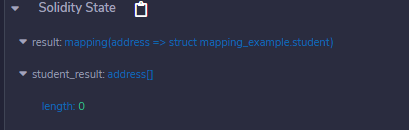As the mapping is created let’s try to add some values to the mapping for better understanding.

Example: In the below example, the contract mapping_example defines a structure, mapping is created and values are added to the mapping.

## Solidity

 `// Solidity program to ` `// demonstrate adding ` `// values to mapping` `pragma solidity ^0.4.18; `   `// Creating contract` `contract` `mapping_example {` `    `  `    ``//Defining structure ` `    ``struct` `student {`   `        ``//Declaring different ` `        ``// structure elements` `        ``string name;` `        ``string subject;` `        ``uint8 marks;` `    ``}`   `    ``// Creating mapping` `    ``mapping (` `      ``address => student) result;` `    ``address[] ``public` `student_result;` `    `  `    ``// Function adding values to ` `    ``// the mapping` `    ``function adding_values() ``public` `{` `        ``var student ` `          ``= result[0xDEE7796E89C82C36BAdd1375076f39D69FafE252];`   `        ``student.name = ``"John"``;` `        ``student.subject = ``"Chemistry"``;` `        ``student.marks = 88;` `        ``student_result.push(` `          ``0xDEE7796E89C82C36BAdd1375076f39D69FafE252) -1;`   `    ``}` `    `  `}`

Output :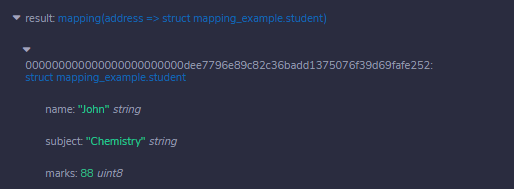### Getting values

We have added values to the mapping, to retrieve the values we have to create a function that returns the values added to the mapping.

Example: In the below example, the contract mapping_example defines a structure, mapping is created, values are added to the mapping, and values are retrieved from the mapping.

## Solidity

 `// Solidity program to` `// demonstrate retrieve` `// values from the mapping`   `pragma solidity ^0.4.18; ` ` `  `contract` `mapping_example {` `    `  `    ``// Defining Structure` `    ``struct` `student {`   `        ``// Declaring different data types` `        ``string name;` `        ``string subject;` `        ``uint8 marks;` `    ``}` `    `  `    ``// Creating mapping` `    ``mapping (` `      ``address => student) result;` `    ``address[] student_result;` `   `  `    ``// Function adding values to the mapping` `    ``function adding_values() ``public` `{` `        ``var student ` `          ``= result[0xDEE7796E89C82C36BAdd1375076f39D69FafE252];`   `        ``student.name = ``"John"``;` `        ``student.subject = ``"Chemistry"``;` `        ``student.marks = 88;` `        ``student_result.push(` `          ``0xDEE7796E89C82C36BAdd1375076f39D69FafE252) -1;`   `    ``}` `    `  `     ``// Function to retrieve ` `     ``// values from a mapping` `     ``function get_student_result(` `     ``) view ``public` `returns (address[]) {` `        ``return` `student_result;` `    ``}` `}`

Output :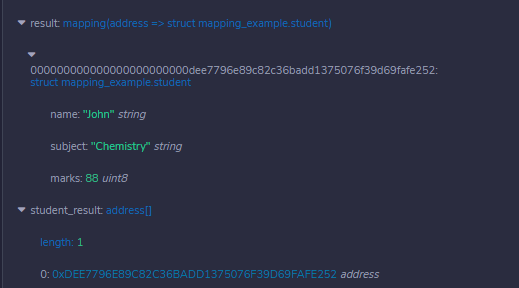### Counting Mappings

Mappings can be counted so that we can know how many values are stored in mapping.

Example: In the below example, the contract mapping_example defines a structure, mapping is created, values are added to the mapping, and values are retrieved from the mapping.

## Solidity

 `// Solidity program to` `// count number of ` `// values in a mapping` `pragma solidity ^0.4.18; ` ` `  `contract` `mapping_example {` `    `  `    ``// Defining structure` `    ``struct` `student {`   `        ``// Declaring different` `        ``// structure elements` `        ``string name;` `        ``string subject;` `        ``uint8 marks;` `    ``}` `    `  `    ``// Creating mapping` `    ``mapping (address => student) result;` `    ``address[] student_result;` `    `  `    ``//Function adding values to the mapping` `    ``function adding_values() ``public` `{` `        ``var student` `          ``= result[0xDEE7796E89C82C36BAdd1375076f39D69FafE252];`   `        ``student.name = ``"John"``;` `        ``student.subject = ``"Chemistry"``;` `        ``student.marks = 88;` `        ``student_result.push(` `          ``0xDEE7796E89C82C36BAdd1375076f39D69FafE252) -1;`   `    ``}` `    `  `     ``// Function to retrieve ` `     ``// values from the mapping` `     ``function get_student_result(` `     ``) view ``public` `returns (address[]) {` `        ``return` `student_result;` `    ``}`   `    ``// Function to count number ` `    ``// of values in a mapping` `    ``function count_students(` `    ``) view ``public` `returns (uint) {` `        ``return` `student_result.length;` `    ``}` `}`

Output :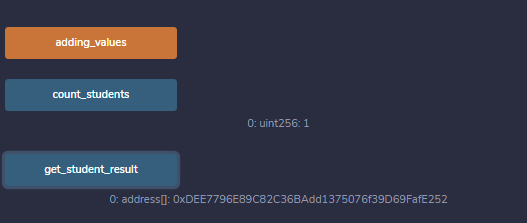Nested Mappings

If you need to keep track of multiple relationships, use nested mapping. Nested mapping is similar to regular mapping, but it has a different syntax.

Syntax:

`mapping(key => mapping(key => value)) <access specifier> <name>;`

Example : In the below example , nested mapping created for electing the manager for an organization. Employees used their Employee ID and Voting choice to cast their vote. For knowing the voting status of the Employees ,You can use their Employee ID and Employee Address.

## Solidity

 `// SPDX-License-Identifier: GPL-3.0` `pragma solidity ^0.8.16;` `/// @title A contract for demonstrating Nested Mapping` `/// @author Jitendra Gangwar` `/// @notice For now, this contract defining the Voting choice of the Employees for electing their Manager` `contract` `NestedMapping{` `    ``//Nested Mapping` `    ``mapping(uint => mapping(address => ``bool``)) voteToManager;` `    ``//function for Vote Registration of the Employees` `    ``function voteRegistration(uint _empId, ``bool` `_voteChoice) external {` `        ``voteToManager[_empId][msg.sender] = _voteChoice;` `    ``}` `    ``//function for knowing the Voting Status of the Employees` `    ``function getVoteStatus(uint _empId, address _empAddr) external view returns(``bool``){` `            ``return` `voteToManager[_empId][_empAddr];` `        ``} ` `    ``}`

Output :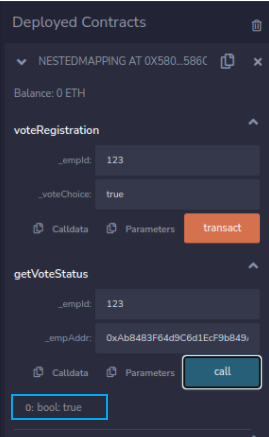Output

Whether you're preparing for your first job interview or aiming to upskill in this ever-evolving tech landscape, GeeksforGeeks Courses are your key to success. We provide top-quality content at affordable prices, all geared towards accelerating your growth in a time-bound manner. Join the millions we've already empowered, and we're here to do the same for you. Don't miss out - check it out now!

Previous
Next